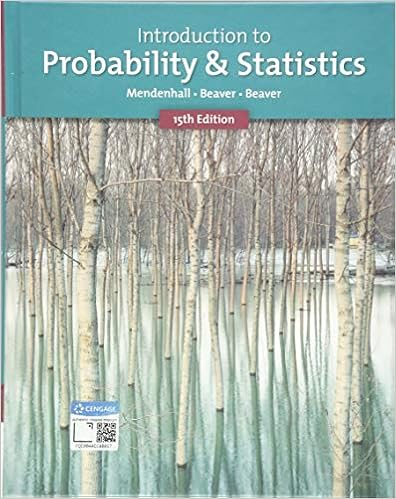# Perry purchased 100 shares of ferro inc common stock

• Test Prep
• 8
• 92% (12) 11 out of 12 people found this document helpful

This preview shows page 4 - 6 out of 8 pages.

##### We have textbook solutions for you!
The document you are viewing contains questions related to this textbook.The document you are viewing contains questions related to this textbook.
Chapter 4 / Exercise 16
Introduction to Probability and Statistics
Beaver/MendenhallExpert Verified
15.Perry purchased 100 shares of Ferro, Inc. common stock for \$25 per share one year ago. During the year, Ferro, Inc. paid cash dividends of \$2 per share. The stock is currently selling for \$30 per share. If Perry sells all of his shares of Ferro, Inc. today, what rate of return would he realize?A)28%B) 42%C) 1%D) 100%P0= \$25P1 = \$30D1 = \$2Return = (Capital gain + Dividend) / P0Return = (30-25+2)/25 = 7/25 = 28%
16.The larger the difference between an assetʹs worst outcome from its best outcome, the higher the risk of the asset.
17.The lower the coefficient of variation, the greater the risk and therefore the higher the expected return.
18.________ probability distribution shows all possible outcomes and associated probabilities for a given event.
19.The expected value, standard deviation of returns, and coefficient of variation for asset A are (See below.)A) 10 percent, 8 percent, and 1.25, respectively.B) 9.33 percent, 8 percent, and 2.15, respectively.C) 9.35 percent, 4.68 percent, and 2.00, respectively.D) 9.35 percent, 2.76 percent, and 0.295, respectively.
##### We have textbook solutions for you!
The document you are viewing contains questions related to this textbook.The document you are viewing contains questions related to this textbook.
Chapter 4 / Exercise 16
Introduction to Probability and Statistics
Beaver/MendenhallExpert Verified
20.Nico bought 100 shares of Cisco Systems stock for \$24.00 per share on January 1, 2002. He received a dividend of \$2.00 per share at the end of 2002 and \$3.00 per share at the end of 2003. At the end of 2004, Nico collected a dividend of \$4.00 per share and sold his stock for \$18.00 per share. What was Nicoʹs realized holding period return?
•••